# GMAT Math : DSQ: Calculating the length of the diameter

## Example Questions

### Example Question #1 : Dsq: Calculating The Length Of The Diameter

What is the circumference of a circle?

(1)The diameter of this circle is 10

(2)The area of this circle is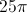Statement (1) ALONE is sufficient, but statement (2) alone is not sufficient.

BOTH statements TOGETHER are sufficient, but NEITHER statement ALONE is sufficient.

Statements (1) and (2) TOGETHER are NOT sufficient.

Statement (2) ALONE is sufficient, but statement (1) alone is not sufficient.

EACH statement ALONE is sufficient.

EACH statement ALONE is sufficient.

Explanation:

The calculation of the circumference is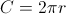. From statement (1) we know that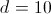. Therefore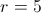, and we can calculate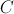using the formula. From statement (2) we know that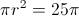. Therefore, and we can calculateusing the formula.

### Example Question #2 : Dsq: Calculating The Length Of The Diameter

Ifandare points on a plane andlies inside the circlewith center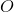and radius 5, doeslie inside circle?

(1) The length of line segment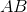is 7.

(2) The length of line segment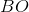is 7.

BOTH statements TOGETHER are not sufficient to answer the question.

EACH statement ALONE is sufficient to answer the question.

Statement 1 ALONE is sufficient to answer the question, but the other statement alone is not sufficient.

Statement 2 ALONE is sufficient to answer the question, but the other statement alone is not sufficient.

BOTH statements TOGETHER are sufficient to answer the question, but NEITHER statement ALONE is sufficient.

Statement 2 ALONE is sufficient to answer the question, but the other statement alone is not sufficient.

Explanation:

(1) The max distance between two points in the circle is twice the length of the

raidus (diameter =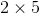=). However,can still be anywhere on the plane

(outside of the circle) as the statement does not indicate otherwise. Therefore, this statement is insuffieicent.

(2) The length of the line segment fromtois greater than the radius of the circle. Thus,must be outside of the circle. This statement is sufficient.

### Example Question #3 : Dsq: Calculating The Length Of The Diameter

Find the diameter of circle B.

I) Circle B has a circumference of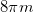.

II) Circle B has an area of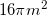.

Neither statement is sufficient to solve the question. More information is needed.

Statement 2 is sufficient to solve the question, but statement 1 is not sufficient to solve the question.

Both statements taken together are sufficient to solve the question.

Each statement alone is enough to solve the question.

Statement 1 is sufficient to solve the question, but statement 2 is not sufficient to solve the question.

Each statement alone is enough to solve the question.

Explanation:

We are given the area and circumference of a circle and asked to find the diameter.

Given the following equations: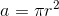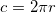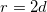We can see that having either area or circumference will allow us to find the radius and in turn the diameter.

Thus, either statement is sufficient by itself.

### Example Question #4 : Dsq: Calculating The Length Of The Diameter

Find the diameter of circle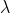I) The area ofofis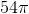.

II) An arc making up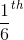ofis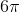.

Both statements together are needed to answer the question.

Statement II is sufficient to answer the question, but Statement I is not sufficient to answer the question.

Statement I is sufficient to answer the question, but Statement II is not sufficient to answer the question.

Either statement alone is sufficient to answer the question.

Either statement alone is sufficient to answer the question.

Explanation:

I) Gives us a portion of the area of the circle. From this we can find the total area and solve for the radius. Then we can double our answer to find the diameter.

II) Gives us a portion of the circumference. From this we can find the total circumference and work our way back to the radius and then the diameter.

### Example Question #5 : Dsq: Calculating The Length Of The Diameter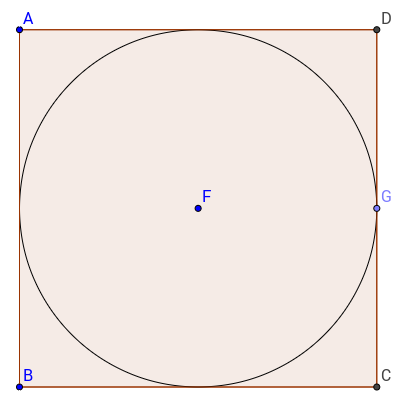The circle of centeris inscribed in square. What is the diameter of the circle?

(1) The ratio of the diameter to the circumference of the circle is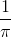.

(2) The area of squareis.

Statement 2 alone is sufficient.

Each statement alone is sufficient.

Both statements together are sufficient.

Statement 1 alone is sufficient.

Statments 1 and 2 together are not sufficient.

Statement 2 alone is sufficient.

Explanation:

To find the diameter of the circle we would need information about the square or about the circle itself.

Statement 1 gives us a ratio of the diameter to the circumference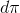of the circle.

If we write the equation it is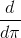or.

Therefore, for all circles, the ratio of the diameter to the circumference will be.

This statement is not helping.

Statement 2 on the other hand gives us the area of the square and therefore allows us to calculate the side of the circle, which is the same as the diameter.

Hence, statement 2 alone is sufficient.

Tired of practice problems?

Try live online GMAT prep today.